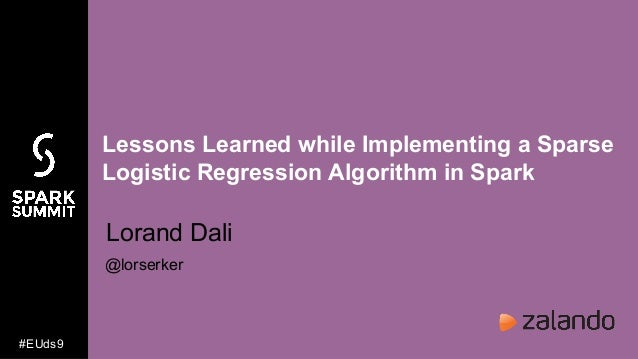Successfully reported this slideshow.Upcoming SlideShare
×

# Lessons Learned while Implementing a Sparse Logistic Regression Algorithm in Apache Spark with Lorand Dali

883 views

Published on

This talk tells the story of implementation and optimization of a sparse logistic regression algorithm in spark. I would like to share the lessons I learned and the steps I had to take to improve the speed of execution and convergence of my initial naive implementation. The message isn’t to convince the audience that logistic regression is great and my implementation is awesome, rather it will give details about how it works under the hood, and general tips for implementing an iterative parallel machine learning algorithm in spark. The talk is structured as a sequence of “lessons learned” that are shown in form of code examples building on the initial naive implementation. The performance impact of each “lesson” on execution time and speed of convergence is measured on benchmark datasets. You will see how to formulate logistic regression in a parallel setting, how to avoid data shuffles, when to use a custom partitioner, how to use the ‘aggregate’ and ‘treeAggregate’ functions, how momentum can accelerate the convergence of gradient descent, and much more. I will assume basic understanding of machine learning and some prior knowledge of spark. The code examples are written in scala, and the code will be made available for each step in the walkthrough.

Published in: Data & Analytics
• Full Name
Comment goes here.

Are you sure you want to Yes No• Be the first to comment

### Lessons Learned while Implementing a Sparse Logistic Regression Algorithm in Apache Spark with Lorand Dali

1. 1. Lessons Learned while Implementing a Sparse Logistic Regression Algorithm in Spark Lorand Dali @lorserker #EUds9
2. 2. You don’t have to implement your own optimization algorithm* *unless you want to play around and learn a lot of new stuff
3. 3. Use a representation that is suited for distributed implementation
4. 4. Logistic regression definition weights Feature Vector Prediction Loss Weight update Derivative of loss Gradient
5. 5. Logistic regression vectorized weights Predictionsfeatures examples Dot products
6. 6. How to compute the gradient vector
7. 7. Computing dot products and predictions
9. 9. weights Partitions Examples Predictions Array[Double] RDD[(Long, Double)] Seq[(Int, Double)] RDD[(Long, Seq[(Int, Double)])] Column index Feature value row index Map[Int, Double]
10. 10. Gradient Array[Double] Prediction minus label Transposed data matrix RDD[(Long, Double)] RDD[(Long, Seq[(Int, Double)])]
11. 11. Experimental dataset - avazu click prediction dataset (sites) - 20 million examples - 1 million dimensions - we just want to try it out https://www.csie.ntu.edu.tw/~cjlin/libsvmtools/datasets/binary.html#avazu
12. 12. Learning curve
13. 13. time per iteration AWS EMR Cluster 5 nodes of m4.2xlarge
14. 14. Use a partitioner to avoid shuffles
15. 15. We have two joins in our code
16. 16. Why is the join expensive + * * * Needs shuffle No shuffle
17. 17. Using a custom partitioner
18. 18. time per iteration
19. 19. Try to avoid joins altogether
20. 20. Gradient descent without joins dimension
21. 21. time per iteration
22. 22. Use aggregate and treeAggregate
23. 23. Gradient (part) Features Examples Tree aggregate Comb OP Seq op
24. 24. Seq Op
25. 25. Comb Op
26. 26. time per iteration
27. 27. If you can’t decrease the time per iteration, make the iteration smaller
28. 28. Mini batch gradient descent
29. 29. Learning curve still OK
30. 30. time per iteration
31. 31. time per iteration
32. 32. If time per iteration is minimal, try to have fewer iterations
33. 33. Find a good initialization for the bias - Usually we initialize weights randomly (or to zero) - But a careful initialization of the bias can help (especially in very unbalanced datasets) - We start the gradient descent from a better point and can save several iterations
34. 34. Learning curve before bias init
35. 35. Learning curve after bias init
36. 36. Try a better optimization algorithm to converge faster
37. 37. ADAM - converges faster - combines ideas from: gradient descent, momentum and rmsprop - basically just keeps moving averages and makes larger steps when values are consistent or gradients are small - useful for making better progress in plateaus